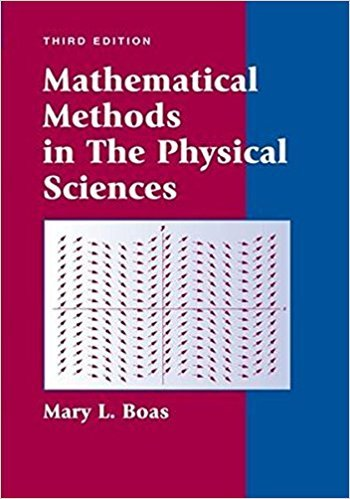×
×

# Solutions for Chapter 7: Fourier Series and Transforms## Full solutions for Mathematical Methods in the Physical Sciences | 3rd Edition

ISBN: 9780471198260Solutions for Chapter 7: Fourier Series and Transforms

Solutions for Chapter 7
4 5 0 255 Reviews
22
0
##### ISBN: 9780471198260

This textbook survival guide was created for the textbook: Mathematical Methods in the Physical Sciences, edition: 3. Chapter 7: Fourier Series and Transforms includes 36 full step-by-step solutions. This expansive textbook survival guide covers the following chapters and their solutions. Mathematical Methods in the Physical Sciences was written by and is associated to the ISBN: 9780471198260. Since 36 problems in chapter 7: Fourier Series and Transforms have been answered, more than 8031 students have viewed full step-by-step solutions from this chapter.

Key Physics Terms and definitions covered in this textbook
• //

parallel

• any symbol

average (indicated by a bar over a symbol—e.g., v¯ is average velocity)

• °C

Celsius degree

• °F

Fahrenheit degree

×